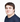Niilo Jaba

# How Does SceneSteam Triangulate 3D Geometry?

Back to blog
November 6, 2018

In computer graphics, there are multiple different ways to represent 3D geometry. Basically, these can be broken down to three different groups: volumetric representations with a set of polyhedrons, surface representations with a set of polygons and point clouds with a set of points.

All of these have advantages and limitations for different use cases. In real-time rendering, triangle-based surface meshes have been the dominating geometrical representation and consumer graphics hardware is optimized for rendering large amounts of triangles as fast as possible.

SceneStream's automatic 3D content optimization pipeline supports a variety of geometrical representations as input. This input data is transformed into an intermediate volumetric representation, which is more suitable for certain geometrical works. Finally, we extract triangle surface mesh out of this volumetric representation.

## Triangulation Scheme

Our triangle surface meshes ensure constrained Delaunay triangulation. In Delaunay triangulation all triangles must satisfy Delaunay condition, i.e., the circumcircles of all triangles have empty interior without any vertices inside. This condition can be generalized to any dimension by replacing triangle with other simplex and circumcircle with the corresponding circum-hypersphere. For two dimensional case, there is also an alternative way to verify this condition, by looking at triangle pair that is connected with a shared edge. When the sum of edge opposite angles is less than or equal to 180 degrees, the Delaunay condition is satisfied. When the triangle pair doesn’t meet the Delaunay condition, it’s possible to correct it by flipping the edge (see Figure 1).

Figure 1: One quadrilateral with two different triangulations. On the left, the sum of the edge opposite angles is over 180 degrees. On the right, the sum of edge opposite angles is less than 180 degrees. Only right one satisfies the Delaunay condition.

## Why Delaunay Triangulation?

Delaunay triangulation has many useful properties, one being that it maximizes the minimum angle of all the angles of the triangles in the triangulation. This highlighted property is important because it’s directly related to minimizing sliver triangles, which are triangles with one or two extremely acute angles. These thin/long shaped triangles are generally not desired for multiple different reasons.

For instance, the cross product between two triangle edges is a very frequent operation, which gives a vector that points towards the triangle’s surface normal. With sliver triangles, these vectors can be nearly parallel or have near zero magnitudes, which can cause numerical stability issues with floating point math.

One common operation is to compute vertex normals for the mesh, by summing all of the connected triangle surface normals for the vertex and weighting it with the surface area of the triangle. This area weighted triangle surface normal is actually given directly by the previously mentioned cross product operation between triangle edges, so the numerical stability issues are already a concern here. Additionally, sliver triangles can cause uneven triangle area distribution, which in some worst cases can lead to nearly degenerate triangles with almost zero area. This can screw up the weighting process and result in badly inaccurate vertex normals, as seen in Figure 2.

Sliver triangles can also reduce the real-time rendering performance. Modern graphics hardware rasterizer works by processing multiple pixels at once. Some of the pixels in these batches are not actually covered by the triangle and end up being discarded from the rendered output. Sliver triangles have usually less coverage on the screen than other triangles, which will result in more discarded processed pixels.

Figure 2: Two triangulations of the same 3D geometry, with vertex normals that are computed from the triangles. On the left triangulation is arbitrary and causes nearly degenerate triangle with a shape similar to the T-vertex situation. On the right, Delaunay triangulation is applied, which ends up flipping the degenerate triangle and therefore fixing vertex normal computation.

## Results

Here in Figure 3, it can be seen how the output looks like when Delaunay triangulation is forced off from Umbra. There exists a lot of undesired triangles, that can be seen from the wireframes. When Delaunay triangulation is enabled, the triangulation looks a lot more natural and balanced. Note that there are some triangles that seem unnecessary. We split our output into multiple streaming blocks, which require some additional triangles around the block boundary.

Figure 3: On the left is an extracted triangle mesh with arbitrary triangulation. On the right, similarly extracted triangle mesh where Delaunay condition is enforced for the triangulation.